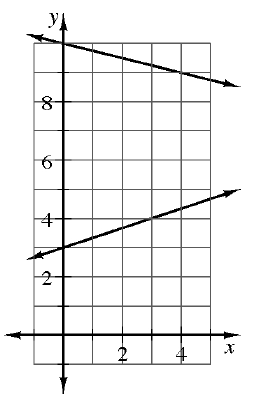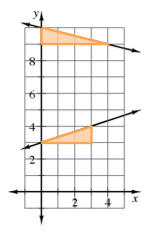### Home > CAAC > Chapter 11 > Lesson 11.2.1 > Problem11-82

11-82.

Examine the two lines graphed below. Will these two lines intersect? Find the equation of each line and test your prediction.Find the slopes of each line by making slope triangles for each.
Look at the graph to determine the $y$-intercept for each line.Use the Equal Values Method to solve the system.
Is there a solution?

Use the eTool below to test your prediction.
Click on the link at right for the full eTool version: